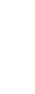•
•
•
•

This is the 3rd article of our series “Binary Options Trading”

In the previous articles, I’ve shown you how to trade binary options and a simple binary options trading strategy using Bollinger Bands.

In this article, we’ll take it to the next level, so let’s get started….

The main difference between “regular” Binary Options and Nadex Call Spreads is this:

When trading Binary Options, you are simply choosing whether a market is trading above or below a certain level.

In order to trade this Binary Option, you pay between \$0 and \$100.

In this example, let’s say you’re paying \$40.

If you are right, you’re getting \$100 back, i.e. you made \$100 – \$40 = \$60.
If you are wrong, you get \$0 back, i.e. you lost \$40

Pretty straightforward.

• You don’t only have ONE strike price – you know have TWO, and they are called a FLOOR and a CEILING.
• And it’s not an “all or nothing:” As long as prices are trading above your entry, you can still make money.

Here’s an example:

Let’s say the Dow Jones is at 26,900 right now.

And we want to trade a Nadex Call Spread with a floor of 26,800 and a ceiling of 27,200.

The price of this call spread will be a little more than \$100 since there’s some premium in the spread.

So let’s say we have to pay \$120 for the spread.

If the Dow closes above the ceiling, i.e. 27,200, we would get \$400 back.
So we make \$400 – \$120 = \$280.

And if the Dow closes below the floor, i.e. 26,800, we would get \$0 back.
So we lose the amount we paid, i.e. \$120.

But here’s the cool thing:

For every point that the Dow closes above the floor, we make \$1.

So if the Dow closes at 27,000, it would be 200 points above the ceiling. This means that we’re getting \$200 back. We paid \$120 for the spread and we get \$200 back, i.e. we made \$80.

As you can see, there are many advantages to call spreads vs. “regular” Binary Options:

• It’s not an “all or nothing” scenario: As long as prices close above our entry price, we make money.
• Our loss is still capped to the amount we paid for the spread. In this example, it’s \$120.
• Our profit is also capped, but it’s no longer \$100. In this example, it’s \$400, which allows us to create a trade with a better risk/reward ratio.Learn to trade stocks & options in 15 minutes a day

Here’s where the magic happens:

When we combine Call Spreads with Binary Options, we’re getting a very nice risk/reward ratio!

Here’s an example:

Here’s the risk graph for this spread:

And let’s say that at the same time, we are selling a Binary Option with a strike price of 26,900 for \$54.

This means that we get \$100 when the Dow closes below 26,900, so we keep the \$54.

And if the Dow closes above 26,900, we get nothing back, so we lose \$46.

I know… this can make your head spin but bear with me.

This is an advanced strategy, and it’s VERY cool!

So here’s the risk graph for the Binary Option:

Can you see what’s happening?

We’re making money on the Nadex Call Spread when prices move above 26,900.

And we are making money on the Binary Option when prices move below 26,900.

We make money when prices move above 26,900 and we make money when prices move below 26,900.

Let that sink in for a moment!
How cool is this?

Yes, there’s a possibility that we can lose money. Let me show you the combined risk graph:

As you can see, there’s a small area when we lose money:

If the Dow closes at exactly 26,900, we would lose \$111.

But how likely is that?

If we enter this trade in the morning when the Dow is at 29,600 – how likely do you think it is that the Dow CLOSES at this exact level?

Not very much, right?

Here are the possible scenarios:

• As long as the Dow closes between 26,900 and 27,000, we lose a little bit of money.
• If the Dow closes above 27,000, we are making money, up to \$188.
• If the Dow closes between 26,800 and 26,900, we are making money, up to \$88.
• If the Dow closes below 26,800, we lose \$7.

As you can see, in most scenarios we’re making money.

The worst-case scenario is when the Dow closes exactly where it opened, which is very unlikely.

The Next Step

That’s why I put together a more in-depth training for you. And the best: It’s free.

And if you missed any of the previous articles, just click on one links below to read it now:

How Binary Options Work – Can You Make Money With Binary Options?